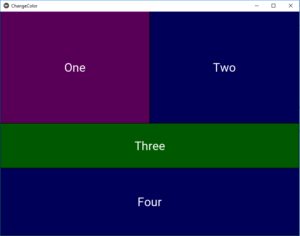# Change button Color in Kivy

• Last Updated : 27 Feb, 2020

Kivy is a platform independent GUI tool in Python. As it can be run on Android, IOS, Linux and Windows, etc. It is basically used to develop the Android application, but it does not mean that it can not be used on Desktops applications.

In this article, we will learn about how to change the button color in kivy. There is a property named background_color which is used to change the color of the button in kivy python .

background_color – The background-color kivy property sets the background color of an element. It is specified as a single color value.

Syntax: background_color: 1, 0, 0, 1

Note: By default the color of button is black (little grey) if you want to change it then we use this property.and it only takes the value between 0 to 1 any other value given will lead to misbehaving of program.

Basic Approach to follow while changing button color:
1) import kivy
2) import kivyApp
3) import all needed
4) set minimum version(optional)
6) Add buttons at set their colors
6) Extend the class
7) Return layout
8) Run an instance of the class

Kivy Tutorial – Learn Kivy with Examples.

Now below is the code how can you change the color of button:

Code 1#

 `def` `build(``self``):  ` `        ``# use a (r, g, b, a) tuple  ` `        ``btn ``=` `Button(text ``=``"Push Me !"``,  ` `        ``font_size ``=``"20sp"``,  ` ` `  `                ``# Here you can give the color ` `                ``# The value must be between 0 to 1  ` `                ``# greyish black color ` `                ``background_color ``=``(``1``, ``1``, ``1``, ``1``),   ` ` `  `                ``size ``=``(``32``, ``32``),  ` `                ``size_hint ``=``(.``2``, .``2``),  ` `                ``pos ``=``(``300``, ``250``))  ` ` `  `        ``return` `btn  `

Code #2

 `## Sample Python application demonstrating the  ` `## How to change button color in Kivy. ` `###################################################  ` `  `  `# import kivy module  ` `import` `kivy  ` ` `  `# to choose the colors randomly  ` `# every time you run it shows different color  ` `import` `random  ` ` `  `# this restricts the kivy version i.e  ` `# below this kivy version you cannot  ` `# use the app or software  ` `kivy.require(``"1.9.1"``)  ` `     `  `# base Class of your App inherits from the App class.  ` `# app:always refers to the instance of your application  ` `from` `kivy.app ``import` `App  ` `     `  `# creates the button in kivy  ` `# if not imported shows the error  ` `from` `kivy.uix.button ``import` `Button  ` ` `  `# BoxLayout arranges children in a vertical or horizontal box.  ` `# or help to put the children at the desired location.  ` `from` `kivy.uix.boxlayout ``import` `BoxLayout  ` ` `  `# declaring the colours you can use directly also  ` `red ``=` `[``1``, ``0``, ``0``, ``1``]  ` `green ``=` `[``0``, ``1``, ``0``, ``1``]  ` `blue ``=` `[``0``, ``0``, ``1``, ``1``]  ` `purple ``=` `[``1``, ``0``, ``1``, ``1``]  ` `     `  `# class in which we are creating the button  ` `class` `ChangeColorApp(App):  ` `         `  `    ``def` `build(``self``):  ` `        ``superBox ``=` `BoxLayout(orientation ``=``'vertical'``)  ` ` `  `        ``HB ``=` `BoxLayout(orientation ``=``'horizontal'``)  ` ` `  `        ``# creating the list of defined colors ` `        ``colors ``=` `[red, green, blue, purple]  ` `         `  ` `  `        ``# Changing the color of buttons ` `        ``# here you can see how you can change the color ` `        ``btn1 ``=` `Button(text ``=``"One"``, ` `                    ``# Color of button is changed not default ` `                    ``background_color ``=` `random.choice(colors),  ` `                    ``font_size ``=` `32``,  ` `                    ``size_hint ``=``(``0.7``, ``1``))  ` ` `  `        ``btn2 ``=` `Button(text ``=``"Two"``,  ` `                    ``background_color ``=` `random.choice(colors),  ` `                    ``font_size ``=` `32``,  ` `                    ``size_hint ``=``(``0.7``, ``1``))  ` ` `  `        ``HB.add_widget(btn1)  ` `        ``HB.add_widget(btn2)  ` ` `  `  `  `        ``VB ``=` `BoxLayout(orientation ``=``'vertical'``)  ` ` `  `        ``btn3 ``=` `Button(text ``=``"Three"``,  ` `                    ``background_color ``=` `random.choice(colors),  ` `                    ``font_size ``=` `32``,  ` `                    ``size_hint ``=``(``1``, ``10``))  ` ` `  `        ``btn4 ``=` `Button(text ``=``"Four"``,  ` `                    ``background_color ``=` `random.choice(colors),  ` `                    ``font_size ``=` `32``,  ` `                    ``size_hint ``=``(``1``, ``15``))  ` ` `  `         `  `        ``VB.add_widget(btn3)  ` `        ``VB.add_widget(btn4)  ` ` `  `        ``superBox.add_widget(HB)  ` `        ``superBox.add_widget(VB)  ` ` `  `        ``return` `superBox  ` ` `  `# creating the object root for App class  ` `root ``=` `ChangeColorApp()  ` `     `  `# run function runs the whole program  ` `# i.e run() method which calls the  ` `# target function passed to the constructor.  ` `root.run()  `

Output:My Personal Notes arrow_drop_up
Recommended Articles
Page :